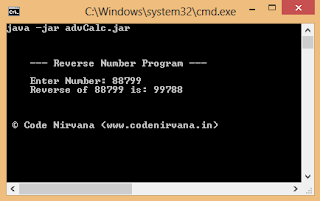# Reverse a Number In Java | With 0 Error Correction

Program Description: Write a program to reverse a number in java.

Source Code:

```import java.util.Scanner;

/* Reverse Numbers Program In JAVA

*/
public class revNumber{

public static void main(String args[]){

System.out.println("--- Reverse Number Program ---\n");
System.out.print("Enter Number: ");
// 0 error correction --important
if(num%10==0){
System.out.print("Reverse of "+num+" is: ");
int temp = num;
for(int i=1;i<=9;i++){
System.out.print(0);
temp=temp/10;
if(temp%10!=0){
break;
}
}
System.out.println(REV(num));
} else{
System.out.println("Reverse of "+num+" is: "+REV(num));
}
}

static int REV(int n){

long Temp,RevNumber=0;
Temp=n;
while (Temp>0)
{
RevNumber=(RevNumber*10)+(Temp%10);
Temp=Temp/10;
}
return  (int) RevNumber;

}
}```

Result:

Program Review:
There are many methods to reverse a number in Java but the most important and commonly asked method to reverse a number is the above explained. This method is important because here we are not using any inbuilt class or function which are generally used to make the code as short as possible, here we are using numeric operations and logic to reverse a input number In java programming.

You might noticed a comment- 0 error correction in the above source code, this correction is the most important step in this program because without this correction your program will definitely failed to reverse a huge list of numbers..... i.e numbers ending with '0'. Lets see how a program without this correction fails to reverse those numbers:-
say if you entered a number= 120 (or any number ending with ), than you will get output= 21
so the above program to reverse a number is free from any error or bug.

If you have any question related to this program or any other program in Java, just leave a reply and we will get back to you as soon as possible!I didn't understand the zero eror correction part in above program. please explainIf any number ends with 0... than count the number of trailing zeros and print before the reverse.Hi, thanks for post, it is clearer now some things for us beginners...please could you answer : what if you want to reverse 00456 ( as 45600)...your program doesn't read first number digit.Thanks for answer